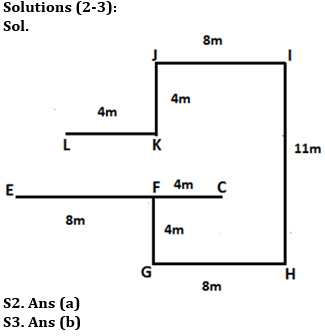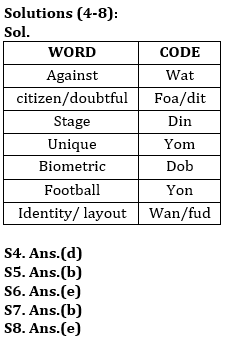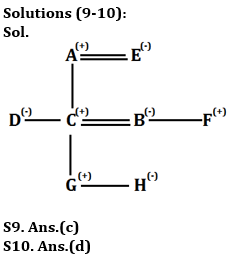Latest Banking jobs   »

# Reasoning Quiz For RBI Grade B Phase 1 2023-20th May

Q1. How many meaningful words can be formed from the 4th, 6th, 7th and 9th letter of a word ‘DOWNGRADE’ by using each letter once in the word?
(a) Two
(b) One
(c) None
(d) Three
(e) More than three

Directions (2-3): Read the following information carefully and answer the given question.
Point E is 8m to the west of Point F which is 4m to the north of Point G. Point I is 11m to the north of Point H which is 8m to the east of Point G. Point K is 4m to the south of Point of J, which is 8m to the west of Point I. Point L is 4m to the west of Point K. Point C is 4m to the east of Point F.

Q2. If point A is 4m to the south of point I, and point B is 4m to the north of point H, then what is the shortest distance between point C and point A?
(a) 5m
(b) 6m
(c) 7m
(d) 11m
(e) Can’t determined

Q3. In which direction point K is with respect to point H?
(a) South-east
(b) North-west
(c) North
(d) West
(e) North-east

Directions (4-8): Answer these questions based on the following information.
In a certain code:
“Stage citizen against doubtful” is coded as “din foa wat dit”
“Citizen doubtful unique” is coded as “foa dit yom”
“Biometric identity layout” is coded as “dob wan fud”
“Football against Biometric” is coded as “yon wat dob”

Q4. What may be the code for “Biometric doubtful”?
(a) foa din
(b) dit yon
(c) yon foa
(d) dob dit
(e) dit foa

Q5. What is the code for “against unique”?
(a) wat foa
(b) wat yom
(c) wat din
(d) dit wat
(e) dit yom

Q6. What is the code for “layout”?
(a) dob
(b) wan
(c) fud
(d) Either dob or wan
(e) Either wan or fud

Q7. What could be the code for “Stage identity”?
(a) wan dit
(b) din wan
(c) foa dob
(d) din wat
(e) dob foa

Q8. What is the code for “football”?
(a) dob
(b) wat
(c) din
(d) yom
(e) yon

Directions (9-10): Study the following information carefully and answer the question given below-
There are eight persons in the family with two married couple. A is father of C. B is sister of F. E is mother of D. F is uncle of H. G is grandson of A. D is sister-in-law of B. C is father of H who is granddaughter of E. D is unmarried. B has two children.

Q9. Who among the following is brother-in-law of C?
(a) A
(b) E
(c) F
(d) G
(e) None of these

Q10. Who among the following is daughter-in-law of A?
(a) H
(b) F
(c) G
(d) B
(e) None of these

Solutions

S1. Ans.(a)
Sol. NEAR, EARN## FAQs

### How many sections are there in the RBI Grade B Phase 1 Exam?

There are 4 sections in the RBI Grade B Phase 1 Exam i.e. English Language, General Awareness, Quantitative Aptitude & Reasoning.

#### Congratulations!Union Budget 2023-24: Free PDF